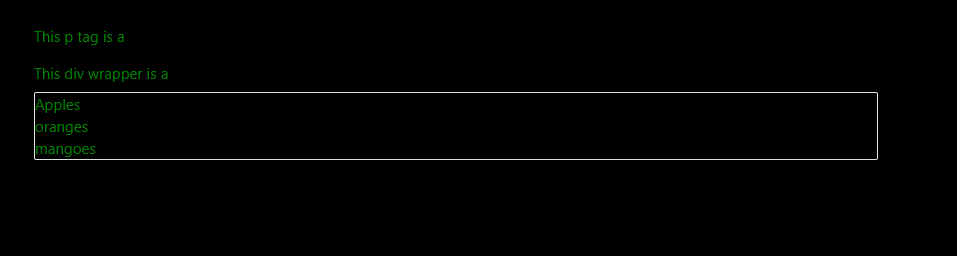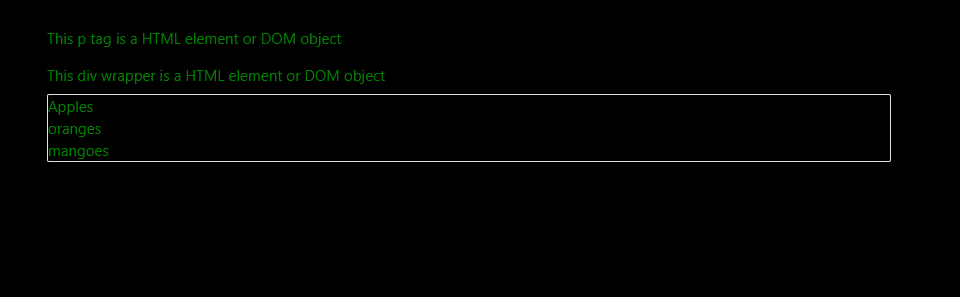# How to check if a JavaScript object is a DOM object?

Here, we are going to learn how to check whether a JavaScript object is a DOM object or not?
Submitted by Siddhant Verma, on December 03, 2019

This method is much easier and also simpler with fewer lines of code. If we look into this, it's something very similar to how we did it before using the for loop. Object.keys() was introduced in ES15 and makes the task of storing all the keys somewhere and then iterating through them simpler.

```const john={
age: 12,
height: 180,
LastName: 'Cena'
}

console.log(john instanceof Array);
console.log(john instanceof Object);
console.log(john instanceof String);
```

Output

```false
true
false
```

john is an object and we can say it's an instance of the object class, therefore it returns true when we check it for an instance of Object and in all other cases for instance of string, array etc it returns false.

```const numbers = [1, 2, 55, 69];

console.log(numbers instanceof Array);
console.log(numbers instanceof Object);
```

Output

```true
true
```

Remember, all arrays are implemented through the object class! Thus the numbers array is both an instance of an array class as it is derived from an array class and also an instance of the object class as the array class itself is derived from an instance class.

```const now = new Date();

console.log(now);
console.log(now instanceof Date);
console.log(now instanceof Object);
console.log(now instanceof String);
```

Output

```Sun Dec 01 2019 09:58:35 GMT+0530 (India Standard Time)
true
true
false
```

Again, the date object is an instance of the date class derived from an object class hence returns true for both and false for other types.

Now that we have a hang of the instanceof operator let's check if a JS object is a DOM object.

In HTML DOM, Element is the general base class for all objects. An Element object represents all HTML elements. Hence to check if an object is a DOM object we'll check it for an instanceof the Element class.

Example: (index.html)

```<html lang="en">

<meta charset="UTF-8">
<meta name="viewport" content="width=device-width, initial-scale=1.0">
<meta http-equiv="X-UA-Compatible" content="ie=edge">
<title>Dom objects</title>
<!-- Compiled and minified CSS -->

<!-- Compiled and minified JavaScript -->
<script src="https://cdnjs.cloudflare.com/ajax/libs/materialize/1.0.0/js/materialize.min.js"></script>

<style>
body {
background: black;
color: green;
}
</style>

<body>
<div class="container">
<p>This p tag is a </p>
<div class="wrapper">
This div wrapper is a
</div>
<ul class="collection list">
<li class="collection-items">Apples</li>
<li class="collection-items">oranges</li>
<li class="collection-items">mangoes</li>
</ul>
</div>
</body>
<script>
const object = {
name: 'John Cena',
age: 45,
profession: 'Wrestler/Actor'
}
const text = document.querySelector('p');
const div = document.querySelector('.wrapper');
const list = document.querySelector('.list');
</script>

</html>
```

OutputWe have a regular JS object and three DOM elements. Remember, everything in JS is wrapped inside an object container so we can't check for an instance of an object. We'll only check for an instance of element class. Let's create a function which does this,

```<script>
function evaluate(obj) {
if (obj instanceof Element) {
const html = ' HTML element or DOM object';
obj.innerText += html;
} else
console.log(`\${obj.name} is a JS Object`);
}
</script>
```

Now let's call this function with different objects passed as parameters,

```<script>
evaluate(object);
evaluate(text);
evaluate(div);
</script>
```

Console: John Cena is a JS Object

OutputThis is how we check is a JavaScript object is a DOM object or a regular JS object?